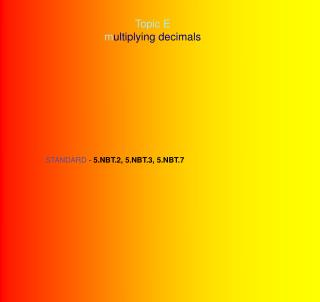DownloadDownload PresentationTopic E m ultiplying decimals

# Topic E m ultiplying decimals

Télécharger la présentation## Topic E m ultiplying decimals

- - - - - - - - - - - - - - - - - - - - - - - - - - - E N D - - - - - - - - - - - - - - - - - - - - - - - - - - -
##### Presentation Transcript

1. Topic E multiplying decimals STANDARD - 5.NBT.2, 5.NBT.3, 5.NBT.7

2. Grade 5 Module 1 Lesson 12 Objective: Multiply a decimal fraction by single- digit whole numbers, including using  estimation to confirm the placement  of the decimal point.

3. MATERIALS NEEDED FOR THIS LESSON Student Student Pages Personal White Boards Sprint A & B Problem Set Exit Ticket Homework Teacher Teacher Pages

4. Fluency Practice(12 minutes)

5. Add Decimals 9 minutes 5.NBT.7 SPRINT A 1 minutes Do as many problems as you can. Do your personal best. Take your mark! Get set! THINK!

6. Add Decimals 9 minutes 5.NBT.7 SPRINT B 1 minutes Do as many problems as you can. Do your personal best. Take your mark! Get set! THINK!

7. Find the Product 3 minutes 5.NBT.7 4 x 2 = 4 x 0.2 = 4 x 0.02 =

8. 5 x 3 = 5 x 0.3 = 5 x 0.03 =

9. 3 x 2 = 3 x 0.03 = 3 x 0.23 = 2 x 0.14 =

10. Application Problem (8 minutes) Patty buys 7 juice boxes a month for lunch. If  one juice costs \$2.79, how much money does  Patty spend on juice each month? Use an area  model to solve.

11. Concept Development (30 minutes)

12. Multipling Decimals 31 x 4 = 3.1 x 4 = 0.31 x 4 = How are these problems  alike?

13. Problem 1 3 tens + 1 one 31 x 4 = 4 12 tens  4 ones answer 120 + 4 = 124

14. Problem 2 3 ones + 1 tenth 3.1 x 4 = 4 12 ones  4 tenths answer 12 + 0.4 = 12.4

15. Problem 3 0.31 x 4 = 3 tenths + 1 hundredths 4 hundredths 4 12 tenths  answer .12 + 0.04 = .124

16. Problem 4 5.1 x 6 = 5 1 tenth x 6 30 6 answer

17. Problem 5 11.4 x 4 = 1 1 4 tenth x 4 4 4 16 4 5 6 answer

18. Problem 6 7.8 x 3 = 7 8 tenth x 3 21 24 2 3 4 answer

19. Problem 7 1248 1.248 12.48 124.8 3.12 x 4 =

20. Problem 8 1610 1.610 16.10 161.0 3.22 x 5 =

21. Problem 9 3.42 x 6 = 2,052 2.052 20.52 205.2

22. 2.932 29.32 293.2 2,932 Problem 10 reasonable answer 0.733 x 4 = 7 tenths + 3 hundredths + 3 thousandths 12 hundredths 28 tenths 12 thousandths area model 4 7 3 3 thousandths x 4 2.8 + 0.12 + 0.012 =2.932 vertical multiplication 28 12 12 2 9 3 2

23. 42,932 4.2932 42.932 429.32 4293.2 Problem 11 reasonable answer 10.733 x 4 = 1 0 7 3 3 thousandths x 4 1 ten + 0 ones + 7 tenths + 3 hundredths + 3 thousandths vertical multiplication 4 40 12 28 12 0 4 0 28 12 12 4 2 9 3 2 40 + 0 + 2.8 + 0.12 + 0.012 = _________________ area model

24. 22,932 2.2932 22.932 229.32 2293.2 Problem 12 reasonable answer 5.733 x 4 = 5 7 3 3 thousandths x 4 5 ones + 7 tenths + 3 hundredths + 3 thousandths vertical multiplication 4 12 20 12 28 20 28 12 12 2 2 9 3 2 20 + 2.8 + 0.12 + 0.012 = _________________ area model

25. Problem Set (10 minutes) Do Your Personal Best (Complete independently!)

26. Student Debrief (10 minutes) How can whole number multiplication help you  with decimal multiplication? How does the area model help you to justify the  placement of the decimal point for the product in 1b?

27. EXIT TICKET (3 minutes) Complete the exit ticket and turn in...

28. HOMEWORK